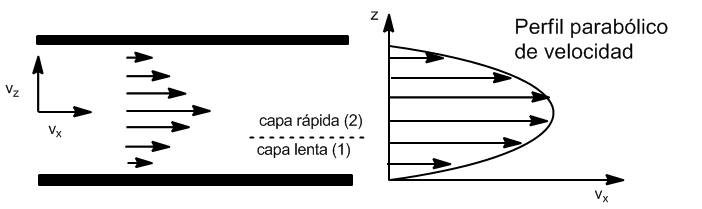Viscosity is the property that characterizes a fluid's resistance to flow. Fluids that flow easily are low in viscosity. Viscosity is represented by $\eta$, and its units are $Ns/m^2$ Consider a fluid that flows between two large, flat, parallel sheets.

Experience tells us that the speed $v_x$ is maximum in the center and zero on the sheets. The horizontal layers of fluid slide over each other, exerting a frictional force that resists displacement.

The force $F_x$ exerted by the slower moving fluid (1) is proportional to the area of the contact surface, A, and the velocity gradient $\frac{dv_x}{dz}$. The constant of proportionality is the viscosity of the fluid $\eta$

\begin{equation} F_y=-\eta A\frac{dv_y}{dx} \end{equation}

This equation is known as Newton's Law of viscosity. The minus sign indicates that the force of viscosity on the faster moving fluid is opposite to the direction of its motion.

By Newton's Third Law, the faster moving fluid exerts a force $\eta A \frac{dv_y}{dx}$ in the positive $z$ direction on the slower moving fluid.Newton's Law fits well for gases and liquids, as long as the speed is not too high. When Newton's Law is fulfilled we have a laminar flow. At very high speeds the flow becomes turbulent and the equation is invalid.

The fluid in which $\eta$ is independent of $dv_x/dz$ is called a Newtonian fluid. In non-Newtonian fluids $\eta$ varies as $dv_x/dz$ does. Most gases are Newtonian, while polymer solutions, colloidal suspensions generally are not, so an increase in flow velocity can change the shape of the (flexible) polymer molecules, facilitating flow by reducing the viscosity.